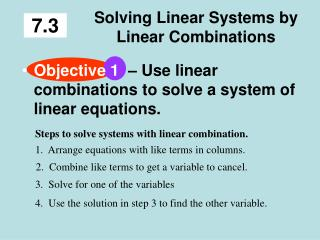DownloadDownload PresentationSolving Linear Systems by Linear Combinations

# Solving Linear Systems by Linear Combinations

Download Presentation## Solving Linear Systems by Linear Combinations

- - - - - - - - - - - - - - - - - - - - - - - - - - - E N D - - - - - - - - - - - - - - - - - - - - - - - - - - -
##### Presentation Transcript

1. 7.3 Solving Linear Systems by Linear Combinations • Objective1 – Use linear combinations to solve a system of linear equations. Steps to solve systems with linear combination. 1. Arrange equations with like terms in columns. 2. Combine like terms to get a variable to cancel. 3. Solve for one of the variables 4. Use the solution in step 3 to find the other variable.

2. Example 3x – 2y = 1 2x + 2y = 4 Combine like terms in columns. The y’s cancel 5x = 5 Divide by 5 5 5 x = 1 Now substitute 1 for x in one of the original equations to solve for y. 2x+ 2y = 4 2(1) +2y = 4 2 + 2y = 4 2y = 2 y = 1 Solution is (1,1)

3. EXAMPLE x + 4y = 23 – x + y = 2 Combine like terms 5y = 25 y = 5 Use y = 5 to solve for x x + 4y = 23 x + 4(5) = 23 x + 20 = 23 x = 3 Solution (3, 5)

4. EXAMPLE Using Multiplication x – y = –5 x + 2y = 4 Nothing Cancels!We must multiply one of the equations by a value to get opposites Multiply one equation by by -1 – x + y = 5 x + 2y = 4 –1(x – y = –5) x + 2y = 4 3y = 9 y = 3 x – y = –5 x – 3 = – 5 Solution (-2, 3) x = – 2